2016 Jeep极致旅行从心启程

许多年来，我们往往习惯性地追逐着“一”：世人眼中的成功，公司登陆纳斯达克新三板、城市黄金地段安家立业……不知不觉间，我们好像忘记了一个道理：在人生面前，成功很小。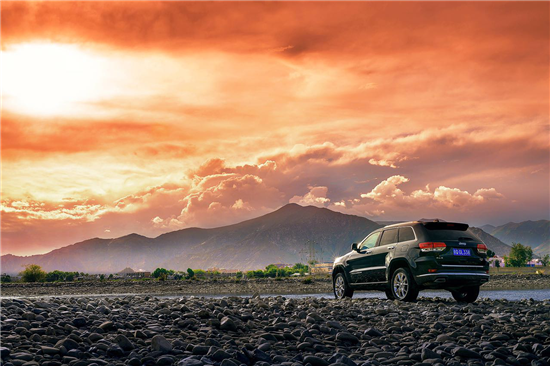无论已经实现何种程度的财务自由，在全球经济增速放缓的大环境下，来到处于一个拐点的时代，如何找到新的入口，连接“过往不再未来没来”的新生态？

Jeep相信，一切答案在每个人的心中。在单纯追逐物质财富的“一”和探享内心丰盈的“多”之间，我们永远站在“多”这边。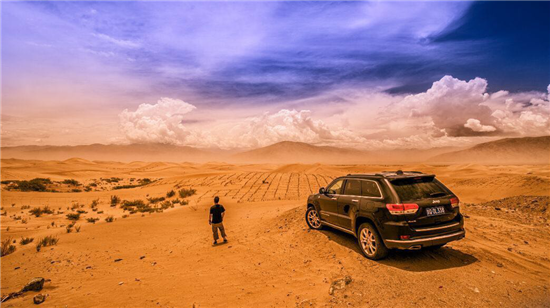2016 Jeep极致旅行：

清心净性、重燃激情、亲子时光、四驱训练，只为从心出发

作为中国专业高端探享体验领导者，Jeep极致旅行2016年携手《中国国家地理》、璞缇客&Feekr等众多业界顶级专业合作伙伴，再次定义一次专业级旅行应该拥有的样子。

探索享受，享受探索。旅行，不是去往一个地方的到此一游，而是满怀信赖倍感安心地踏足未曾领略的风景，为人生收藏全新的阅历，在与自己的对话中探寻未曾发现的可能，为再上征程拓宽心灵之路。在闭关之所跳出三界之外，宽广的道路便在心间悄然开启

很多人去到过香格里拉，但很少人知道，开着传承Jeep 75年专业全路况能力的2016款进口全新大切诺基与进口牧马人，在通过49道悬崖弯路蜿蜒前行之后，在一座尚未天下知的绿色山脉深处，有一处秘境中的秘境。在这里，只有天、地、山、水、身、心，只有云里雾里的风，只有风里雾里的云。这一刻，褪尽浮华，只见自己。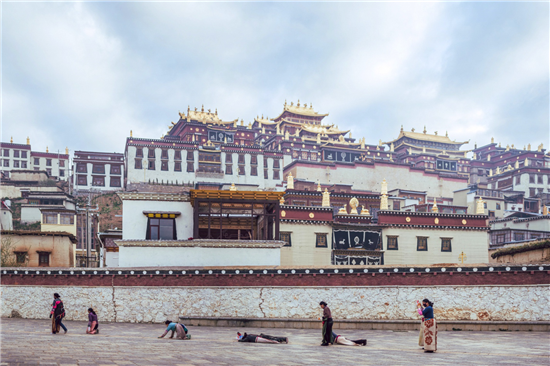再去往松赞林寺的闭关之所，拜访活佛，与高僧打坐冥想同修晨课，尝试一次“跳出三界外不在五行中”的修行；跟随野生动物学者徒步原始森林，探寻国宝滇金丝猴；回到城里，再学习一门2000年前流传下来的藏画技艺，制作全世界仅此一只、专属于你的木碗。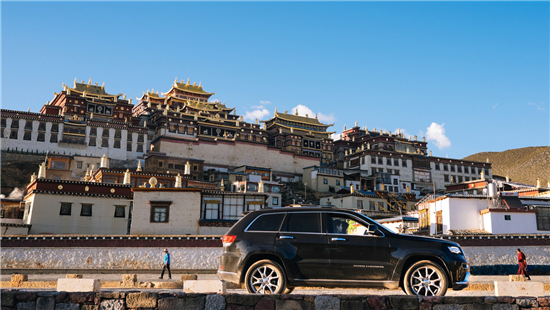先见自己，再见万物，后见众生

是爱情变得平淡了，还是你没有带TA一起走入精彩的世界？

据说结婚七年都得痒，据说很多相处久了的情侣精神世界已经不在同一个纬度，当年心里的轰轰烈烈，渐渐被平淡填满，当时向对方开放的内心世界，渐渐被时间锁上了边界。但是承认吧，不是爱情变得平淡了，而是你没有带TA一起走入精彩的世界。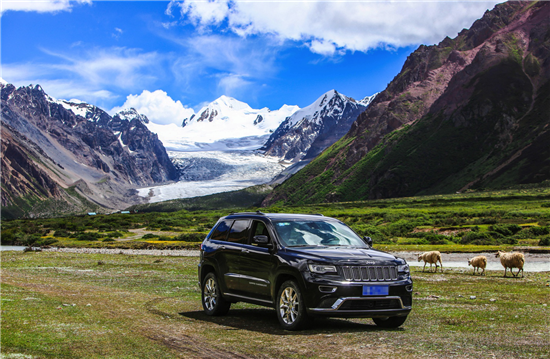这里一半是海水一半是火焰；这里五天行程却蕴含四季风景；这里天苍苍野茫茫风吹草低见牛羊，攀越连绵浩荡的天山山脉，是连接南北新疆的绝美纵贯线；这里有中国第二大草原，天光、云影、湖水、骏马、天鹅和谐相处，构成最美的“天堂草原”；这里，只在一年中特定的时段里准许通车，就好似激情和爱情，都需要定期的维护保养。

跨过10 条大河，去翻越终年积雪的4座冰达坂。历经冰与火的碰撞，壮美和奇险的交错，感受万物和谐，敬畏自然最真实的壮阔。和陪伴你一声的风景，去看遍最原始纯粹的美景，感恩当下的美好。每一段用心营造的亲子时光，都是孩子成长路上的缕缕阳光

你是否总是太忙，回家时孩子已熟睡，起床时孩子已上学？是否只能偶尔带孩子去商场中的游乐场？是否总忘记承诺的全家出游？是否渐渐地，总担心和孩子有代沟？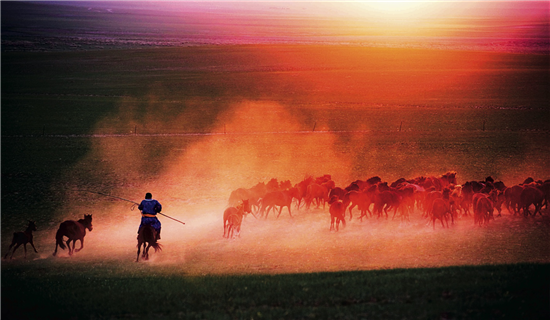坐上送到府上的专业级城市SUV—全新Jeep自由光，自驾前往避暑胜地安吉、莫干山、苏州的10家精品酒店，为和孩子相处的时光，创造更多不一样的回忆：在芳香扑鼻的万亩茶园里以帐篷幕天席地，在青瓦白墙的江南韵调小树、与Hello Kitty为邻的童话之旅、在人民大会堂改造的图书馆里讲述你成长的经历…… 每一段用心营造的亲子时光，都是孩子成长路上的缕缕阳光。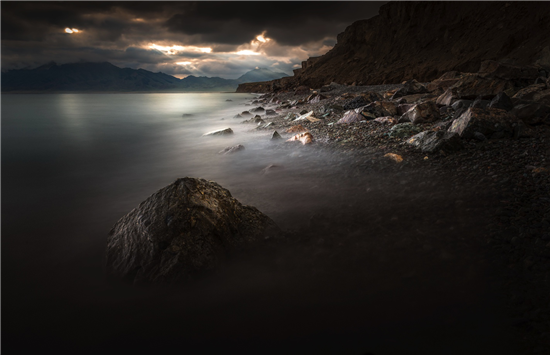比去向远方更重要的，是如何去向远方

空有一颗去向远方的心，却不知道自己驾驶技巧是不是足够？来JeepTrail Rated 专业四驱训练营，去攀爬、去涉水，学习Jeep 与美国军方联合开发的 Trail Rated 4×4课程，助你完成一次次的 “敢走”、“能走”和“想走就走”的洒脱。真正体会Jeep 家族用75年练就的专业能力，在一次次挑战中发现潜能无限的自己。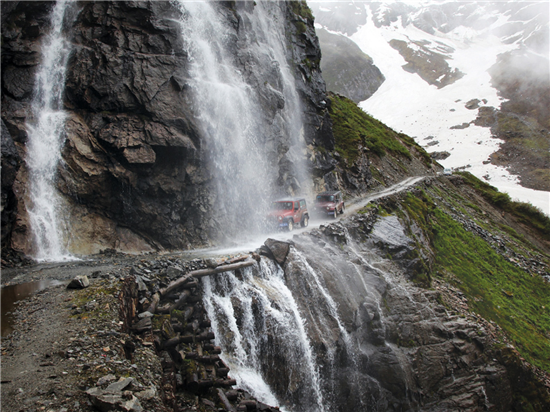在人生面前，成功很小；对5天而言，10年其实很短。2016 Jeep极致旅行7月15日情怀启程，敬候每一位生活探享家。

##### 相关资讯

|分享

•已阅！握爪
•我手滑为你点赞
•128个赞！
•不明觉厉
•阅后既醉
•有钱！任性
•照片太美，我不敢看
•干货！杠杠的
•高大上
•膜拜中！
•请接受我的膝盖
•猫哥/猫妹么么哒
•神吐槽
•我只笑笑不说话
•我想静静
•窒息
##### 资讯相关车系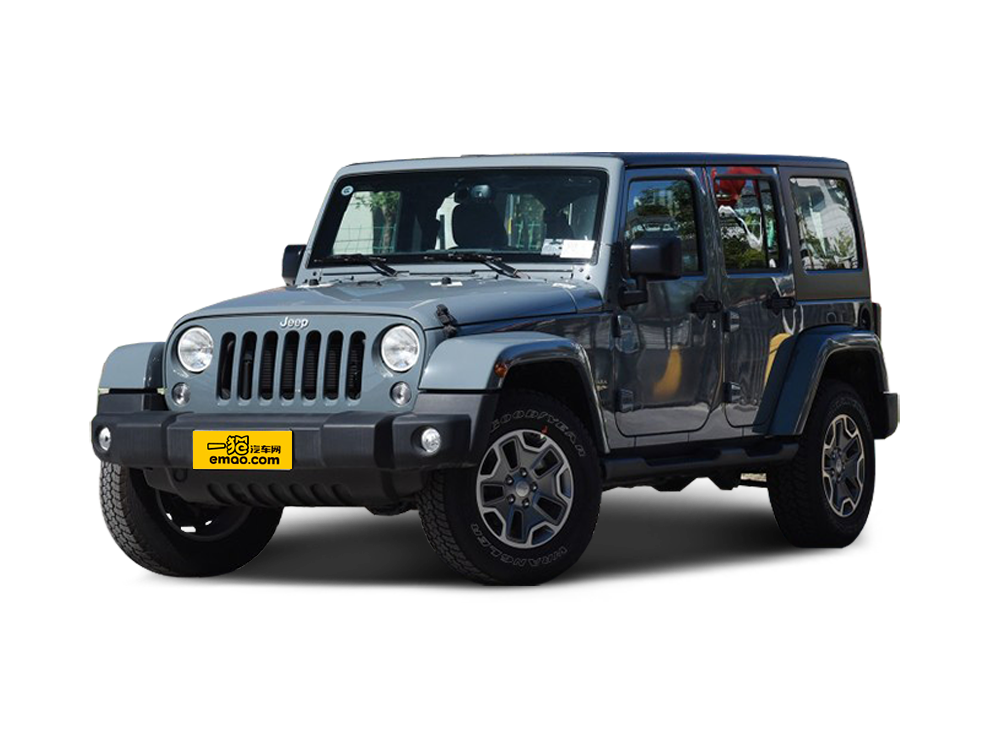##### 热度排行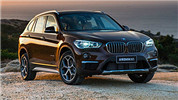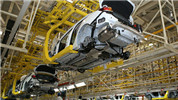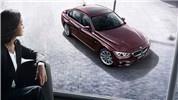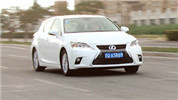CT200h非典型热爱• 阿斯顿·马丁
• 奥迪
• 阿尔法罗密欧
• ALPINA

• 巴博斯
• 宝骏
• 宝马
• 保时捷
• 北汽制造
• 奔驰
• 奔腾
• 本田
• 比亚迪
• 标致
• 别克
• 宾利
• 布加迪
• 北汽威旺
• 北京
• 北汽绅宝
• 北汽幻速
• 北汽新能源
• 宝沃
• 比速汽车
• 北汽道达

• 昌河
• 长安
• 长城
• 长安商用
• 成功汽车
• 长江EV
• 长安轻车型

• 大众
• 道奇
• 东风
• 东风风神
• 东风小康
• 东南
• DS
• 东风风行
• 东风风度
• 东风风光

• 法拉利
• 菲亚特
• 丰田
• 福迪
• 福特
• 福田汽车
• 福汽启腾
• 风诺

• GMC
• 广汽传祺
• 广汽吉奥
• 观致

• 哈飞
• 海格
• 海马
• 华泰
• 黄海
• 恒天
• 红旗
• 哈弗
• 华颂
• 华凯
• 华泰新能源
• 汉腾汽车

• Jeep
• 江淮
• 江铃
• 捷豹
• 金杯
• 九龙
• 吉利汽车
• 金旅
• 金龙
• 江铃集团轻汽
• 江铃集团新能源
• 君马汽车
• 捷途

• 开瑞
• 凯迪拉克
• 科尼赛克
• 克莱斯勒
• KTM
• 卡威
• 凯翼
• 康迪
• 康迪电动汽车集团

• 猎豹汽车
• 兰博基尼
• 劳斯莱斯
• 雷克萨斯
• 雷诺
• 理念
• 力帆
• 莲花汽车
• 林肯
• 铃木
• 陆风
• 路虎
• 路特斯
• 领志
• 领克
• 零跑汽车

• MG
• MINI
• 马自达
• 玛莎拉蒂
• 迈凯伦
• 摩根

• 纳智捷

• 讴歌
• 欧宝
• 欧朗
• 欧拉
• 欧尚汽车

• 帕加尼

• 奇瑞
• 启辰
• 起亚
• 前途
• 庆铃汽车

• 日产
• 荣威
• 瑞麒汽车
• 如虎
• 瑞驰

• 上汽大通
• smart
• 三菱
• 双环
• 双龙
• 斯巴鲁
• 斯柯达
• 萨博
• 思铭
• 赛麟
• SWM斯威汽车

• TESLA
• 泰卡特
• 腾势

• 威麟
• 威兹曼
• 沃尔沃
• 五菱汽车
• 五十铃
• 潍柴英致
• WEY
• 蔚来
• 威马汽车

• 现代
• 雪佛兰
• 雪铁龙
• 西雅特
• 新特汽车
• 小鹏汽车
• 新宝骏

• 野马汽车
• 一汽
• 依维柯
• 英菲尼迪
• 永源
• 驭胜

• 中华
• 中兴
• 众泰
• 知豆
• 之诺
• 正道汽车
• A
• B
• C
• D
• E
• F
• G
• H
• I
• J
• K
• L
• M
• N
• O
• P
• Q
• R
• S
• T
• U
• V
• W
• X
• Y
• Z

• 阿斯顿·马丁
• 奥迪
• 阿尔法罗密欧
• ALPINA

• 巴博斯
• 宝骏
• 宝马
• 保时捷
• 北汽制造
• 奔驰
• 奔腾
• 本田
• 比亚迪
• 标致
• 别克
• 宾利
• 布加迪
• 北汽威旺
• 北京
• 北汽绅宝
• 北汽幻速
• 北汽新能源
• 宝沃
• 比速汽车
• 北汽道达

• 昌河
• 长安
• 长城
• 长安商用
• 成功汽车
• 长江EV
• 长安轻车型

• 大众
• 道奇
• 东风
• 东风风神
• 东风小康
• 东南
• DS
• 东风风行
• 东风风度
• 东风风光

• 法拉利
• 菲亚特
• 丰田
• 福迪
• 福特
• 福田汽车
• 福汽启腾
• 风诺

• GMC
• 广汽传祺
• 广汽吉奥
• 观致

• 哈飞
• 海格
• 海马
• 华泰
• 黄海
• 恒天
• 红旗
• 哈弗
• 华颂
• 华凯
• 华泰新能源
• 汉腾汽车

• Jeep
• 江淮
• 江铃
• 捷豹
• 金杯
• 九龙
• 吉利汽车
• 金旅
• 金龙
• 江铃集团轻汽
• 江铃集团新能源
• 君马汽车
• 捷途

• 开瑞
• 凯迪拉克
• 科尼赛克
• 克莱斯勒
• KTM
• 卡威
• 凯翼
• 康迪
• 康迪电动汽车集团

• 猎豹汽车
• 兰博基尼
• 劳斯莱斯
• 雷克萨斯
• 雷诺
• 理念
• 力帆
• 莲花汽车
• 林肯
• 铃木
• 陆风
• 路虎
• 路特斯
• 领志
• 领克
• 零跑汽车

• MG
• MINI
• 马自达
• 玛莎拉蒂
• 迈凯伦
• 摩根

• 纳智捷

• 讴歌
• 欧宝
• 欧朗
• 欧拉
• 欧尚汽车

• 帕加尼

• 奇瑞
• 启辰
• 起亚
• 前途
• 庆铃汽车

• 日产
• 荣威
• 瑞麒汽车
• 如虎
• 瑞驰

• 上汽大通
• smart
• 三菱
• 双环
• 双龙
• 斯巴鲁
• 斯柯达
• 萨博
• 思铭
• 赛麟
• SWM斯威汽车

• TESLA
• 泰卡特
• 腾势

• 威麟
• 威兹曼
• 沃尔沃
• 五菱汽车
• 五十铃
• 潍柴英致
• WEY
• 蔚来
• 威马汽车

• 现代
• 雪佛兰
• 雪铁龙
• 西雅特
• 新特汽车
• 小鹏汽车
• 新宝骏

• 野马汽车
• 一汽
• 依维柯
• 英菲尼迪
• 永源
• 驭胜

• 中华
• 中兴
• 众泰
• 知豆
• 之诺
• 正道汽车
• A
• B
• C
• D
• E
• F
• G
• H
• I
• J
• K
• L
• M
• N
• O
• P
• Q
• R
• S
• T
• U
• V
• W
• X
• Y
• Z

• 阿斯顿·马丁
• 奥迪
• 阿尔法罗密欧
• ALPINA

• 巴博斯
• 宝骏
• 宝马
• 保时捷
• 北汽制造
• 奔驰
• 奔腾
• 本田
• 比亚迪
• 标致
• 别克
• 宾利
• 布加迪
• 北汽威旺
• 北京
• 北汽绅宝
• 北汽幻速
• 北汽新能源
• 宝沃
• 比速汽车
• 北汽道达

• 昌河
• 长安
• 长城
• 长安商用
• 成功汽车
• 长江EV
• 长安轻车型

• 大众
• 道奇
• 东风
• 东风风神
• 东风小康
• 东南
• DS
• 东风风行
• 东风风度
• 东风风光

• 法拉利
• 菲亚特
• 丰田
• 福迪
• 福特
• 福田汽车
• 福汽启腾
• 风诺

• GMC
• 广汽传祺
• 广汽吉奥
• 观致

• 哈飞
• 海格
• 海马
• 华泰
• 黄海
• 恒天
• 红旗
• 哈弗
• 华颂
• 华凯
• 华泰新能源
• 汉腾汽车

• Jeep
• 江淮
• 江铃
• 捷豹
• 金杯
• 九龙
• 吉利汽车
• 金旅
• 金龙
• 江铃集团轻汽
• 江铃集团新能源
• 君马汽车
• 捷途

• 开瑞
• 凯迪拉克
• 科尼赛克
• 克莱斯勒
• KTM
• 卡威
• 凯翼
• 康迪
• 康迪电动汽车集团

• 猎豹汽车
• 兰博基尼
• 劳斯莱斯
• 雷克萨斯
• 雷诺
• 理念
• 力帆
• 莲花汽车
• 林肯
• 铃木
• 陆风
• 路虎
• 路特斯
• 领志
• 领克
• 零跑汽车

• MG
• MINI
• 马自达
• 玛莎拉蒂
• 迈凯伦
• 摩根

• 纳智捷

• 讴歌
• 欧宝
• 欧朗
• 欧拉
• 欧尚汽车

• 帕加尼

• 奇瑞
• 启辰
• 起亚
• 前途
• 庆铃汽车

• 日产
• 荣威
• 瑞麒汽车
• 如虎
• 瑞驰

• 上汽大通
• smart
• 三菱
• 双环
• 双龙
• 斯巴鲁
• 斯柯达
• 萨博
• 思铭
• 赛麟
• SWM斯威汽车

• TESLA
• 泰卡特
• 腾势

• 威麟
• 威兹曼
• 沃尔沃
• 五菱汽车
• 五十铃
• 潍柴英致
• WEY
• 蔚来
• 威马汽车

• 现代
• 雪佛兰
• 雪铁龙
• 西雅特
• 新特汽车
• 小鹏汽车
• 新宝骏

• 野马汽车
• 一汽
• 依维柯
• 英菲尼迪
• 永源
• 驭胜

• 中华
• 中兴
• 众泰
• 知豆
• 之诺
• 正道汽车
• A
• B
• C
• D
• E
• F
• G
• H
• I
• J
• K
• L
• M
• N
• O
• P
• Q
• R
• S
• T
• U
• V
• W
• X
• Y
• Z